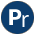#xpath.pl -- Select nodes in an XML DOM

The library xpath.pl provides predicates to select nodes from an XML DOM tree as produced by library(sgml) based on descriptions inspired by the XPath language.

The predicate xpath/3 selects a sub-structure of the DOM non-deterministically based on an XPath-like specification. Not all selectors of XPath are implemented, but the ability to mix xpath/3 calls with arbitrary Prolog code provides a powerful tool for extracting information from XML parse-trees.

- http://www.w3.org/TR/xpath
xpath_chk(+DOM, +Spec, ?Content) is semidet
Semi-deterministic version of xpath/3.
xpath(+DOM, +Spec, ?Content) is nondet
Match an element in a DOM structure. The syntax is inspired by XPath, using () rather than [] to select inside an element. First we can construct paths using / and //:
`//`Term
Select any node in the DOM matching term.
`/`Term
Match the root against Term.
Term
Select the immediate children of the root matching Term.

The Terms above are of type callable. The functor specifies the element name. The element name '*' refers to any element. The name `self` refers to the top-element itself and is often used for processing matches of an earlier xpath/3 query. A term NS:Term refers to an XML name in the namespace NS. Optional arguments specify additional constraints and functions. The arguments are processed from left to right. Defined conditional argument values are:

index(?Index)
True if the element is the Index-th child of its parent, where 1 denotes the first child. Index can be one of:
Var
Var is unified with the index of the matched element.
`last`
True for the last element.
`last` - IntExpr
True for the last-minus-nth element. For example, `last-1` is the element directly preceding the last one.
IntExpr
True for the element whose index equals IntExpr.
Integer
The N-th element with the given name, with 1 denoting the first element. Same as `index(Integer)`.
`last`
The last element with the given name. Same as `index(last)`.
`last` - IntExpr
The IntExpr-th element before the last. Same as `index(last-IntExpr)`.

Defined function argument values are:

`self`
Evaluate to the entire element
`content`
Evaluate to the content of the element (a list)
`text`
Evaluates to all text from the sub-tree as an atom
`text(As)`
Evaluates to all text from the sub-tree according to As, which is either `atom` or `string`.
`normalize_space`
As `text`, but uses normalize_space/2 to normalise white-space in the output
`number`
Extract an integer or float from the value. Ignores leading and trailing white-space
`@`Attribute
Evaluates to the value of the given attribute. Attribute can be a compound term. In this case the functor name denotes the element and arguments perform transformations on the attribute value. Defined transformations are:
number
Translate the value into a number using xsd_number_string/2 from library(sgml).
integer
As `number`, but subsequently transform the value into an integer using the round/1 function.
float
As `number`, but subsequently transform the value into a float using the float/1 function.
string
Translate the value into a Prolog string.
lower
Translate the value to lower case, preserving the type.
upper
Translate the value to upper case, preserving the type.

In addition, the argument-list can be conditions:

Left = Right
Succeeds if the left-hand unifies with the right-hand. If the left-hand side is a function, this is evaluated. The right-hand side is never evaluated, and thus the condition `content = content` defines that the content of the element is the atom `content`. The functions `lower_case` and `upper_case` can be applied to Right (see example below).
`contains(Haystack, Needle)`
Succeeds if Needle is a sub-string of Haystack.
XPath
Succeeds if XPath matches in the currently selected sub-DOM. For example, the following expression finds an `h3` element inside a `div` element, where the `div` element itself contains an `h2` child with a `strong` child.
`//div(h2/strong)/h3`

This is equivalent to the conjunction of XPath goals below.

```   ...,
xpath(DOM, //(div), Div),
xpath(Div, h2/strong, _),
xpath(Div, h3, Result)```

Examples:

Match each table-row in DOM:

`xpath(DOM, //tr, TR)`

Match the last cell of each tablerow in DOM. This example illustrates that a result can be the input of subsequent xpath/3 queries. Using multiple queries on the intermediate TR term guarantee that all results come from the same table-row:

```xpath(DOM, //tr, TR),
xpath(TR,  /td(last), TD)```

Match each `href` attribute in an <a> element

`xpath(DOM, //a(@href), HREF)`

Suppose we have a table containing rows where each first column is the name of a product with a link to details and the second is the price (a number). The following predicate matches the name, URL and price:

```product(DOM, Name, URL, Price) :-
xpath(DOM, //tr, TR),
xpath(TR, td(1), C1),
xpath(C1, /self(normalize_space), Name),
xpath(C1, a(@href), URL),
xpath(TR, td(2, number), Price).```

Suppose we want to select books with genre="thriller" from a tree containing elements `<book genre=...>`

```thriller(DOM, Book) :-
xpath(DOM, //book(@genre=thiller), Book).```

Match the elements `<table align="center">` and ```<table align="CENTER">```:

`    //table(@align(lower) = center)`

Get the `width` and `height` of a `div` element as a number, and the `div` node itself:

`    xpath(DOM, //div(@width(number)=W, @height(number)=H), Div)`

Note that `div` is an infix operator, so parentheses must be used in cases like the following:

`    xpath(DOM, //(div), Div)`
sub_dom(-Index, -Count, +Name, -Sub, +DOM) is nondet[private]
Sub is a node in DOM with Name.
Arguments:
 Count - is the total number of nodes in the content list Sub appears that have the same name. Index - is the 1-based index of Sub of nodes with Name.
count_named_elements(+Content, +Name, -Count) is det[private]
Count is the number of nodes with Name in Content.
nth_element(?N, +Name, -Element, +Content:list) is nondet[private]
True if Element is the N-th element with name in Content.
modifiers(+Modifiers, +I, +Clen, +DOM, -Value)[private]
process_equality(+Left, +Right) is semidet[private]
Provides (very) partial support for XSLT functions that can be applied according to the XPath 2 specification.

For example the XPath expression in , and the equivalent Prolog expression in , would both match the HTML element in .

``` //table[align=lower-case(center)]
 //table(@align=lower_case(center))
 <table align="CENTER">```
text_of_dom(+DOM, +As, -Text:atom) is det[private]
Text is the joined textual content of DOM.